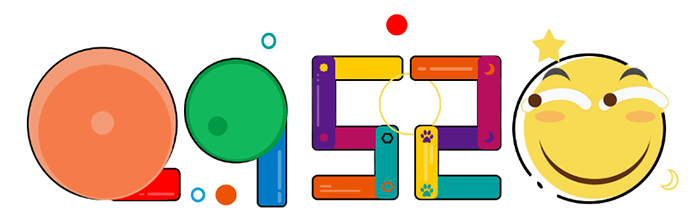# 基于ThinkPHP中App(通信)接口开发封装JSON数据 并读取JSON数据的封装### PHP是世界上最好的语言。

通信数据的标准格式：

code 状态码（200，400等）；
message 提示信息（登录失败，数据返回成功等）；
data 返回数据；

``````public function demo() {
//查询数据库数据
\$json  = \$this->api_rule(\$data,'数据查询成功');
echo \$json;
}``````

PHP开发手机API时，一般返回XML或JSON数据类型的数据，除了要返回从源数据（程序本身需要的数据）外还应附上状态码，以下是一段封装后的数据，它使用JSON格式展现：

``````    /**
* php编写app接口的函数封装
*
* @param  string  \$data    [从数据库中所查出的数据]
* @param  string  \$message [接口的提示信息,解释状态码所使用]
* @param  integer \$code    [状态码]
* @return [type]           [返回json数据类型]
* 接口主要实现了value不为空
* 并且统一将类型转化成字符串
* 将安卓与ios中的关键字进行key的转化,如果是系统关键字，那么我将key添加尾缀 _api进行数组重组
* 一维数组与二维数组通用
*/
public function api_rule(\$data='',\$message='成功',\$code=1)
{
\$all_data=array(
'code'=>\$code,
'message'=>\$message,
);
//数组校验(目的是判断数组几维数组)

//判断是否是数组，并且数组是否大于三维数组
foreach (\$data as \$x => \$y)
{
if(is_array(\$y))
{
foreach (\$y as \$x1 => \$y1)
{
if(is_array(\$y1))
{
echo "函数中不能使用三维以上数组";exit(0);
}
}
}
}
//如果数据不为空的情况下所执行以下代码
if (\$data!=='') {
// app 禁止使用和为了统一字段做的判断,ios的字典中不识别的关键字
\$reserved_words=array('id','title','price','product_title','product_id','product_category','product_number');
foreach (\$reserved_words as \$k => \$v)
{
foreach (\$data as \$ko => \$ko_value)
{
if(is_array(\$ko_value))
{
if (array_key_exists(\$v, \$ko_value))
{
\$keys = \$v.""."_api";
//取出数组中所有的key值
\$keyss = array_keys(\$ko_value);
\$ko_value[\$keys]=\$ko_value[\$v];
unset(\$ko_value[\$v]);
\$data[]=\$ko_value;
array_splice(\$data,0,1);
}

}else{
//检测我数组的key是否存在于这些关键字当中
if (array_key_exists(\$v, \$data))
{
\$keys = \$v.""."_api";
\$data[\$keys]=\$data[\$v];
//取出数组中所有的key值
\$keyss = array_keys(\$data);
\$index = array_search(\$v, \$keyss);
if(\$index !== FALSE){
array_splice(\$data, \$index, 1);
}
}
}
}
}

foreach (\$data as \$key => \$value)
{
if(is_array(\$value))
{
foreach (\$value as \$key12 => \$value12)
{
if(!is_string(\$value12))
{
\$data[\$key][\$key12]=strval(\$value12);
}
//如果我的value是空的情况下，赋予默认值  空
if(empty(\$value12))
{
\$data[\$key][\$key12]="空";
}
}
}else{
if(!is_string(\$value))
{
\$data[\$key]=strval(\$value);
}
//如果我的value是空的情况下，赋予默认值  空
if(empty(\$value))
{
\$data[\$key]="空";
}
}
//如果我的value不是字符串类型，我强转成字符串类型
}

\$all_data['data']=\$data;
}

// 如果是ajax或者app访问；则返回json数据 pc访问直接p出来
return json_encode(\$all_data);
exit(0);
}

``````

## 1 条评论

•文章不错支持一下吧